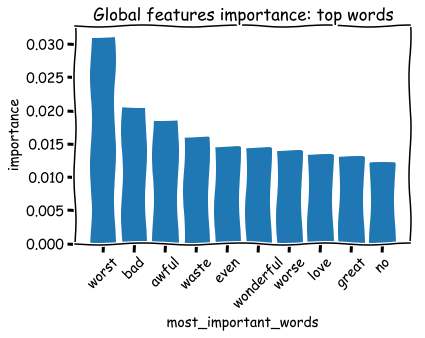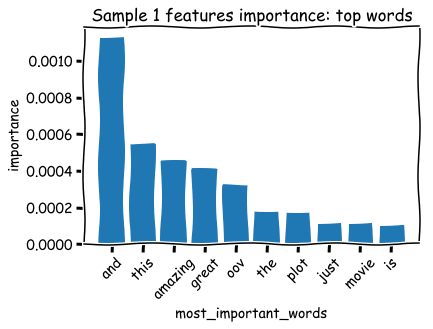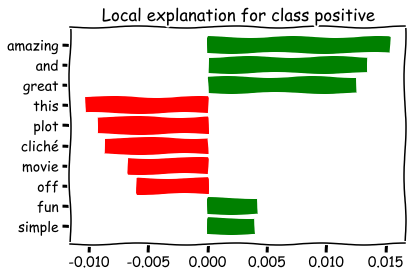# LIME of words: how to interpret your machine learning model predictions

Posted on Mon 07 August 2017

In this blog post I will share experiments on the LIME (Local Interpretable Model-agnostic Explanations) interpretation model. LIME was introduced in 2016 by Marco Ribeiro and his collaborators in a paper called “Why Should I Trust You?” Explaining the Predictions of Any Classifier. The purpose of this method is to explain a model prediction for a specific sample in a human-interpretable way.

It brings a way to investigate a model predictions beyond the sole classification or regression standard metrics. It also may provide explanations to individuals when they are refused a loan or job on the basis of a scoring model, or in other critical situations like the one listed in this very good book.

In order to set the playground I first build a quick model on the IMDB sentiment analysis dataset, which contains movie reviews with an associated sentiment (0 for negative, 1 for positive). Data was downloaded on kaggle, let's have a look at it.

In :
import pandas as pd
df = pd.read_csv('./labeledTrainData.tsv', sep='\t')

Out:
id sentiment review
0 5814_8 1 With all this stuff going down at the moment w...
1 2381_9 1 \The Classic War of the Worlds\" by Timothy Hi...
2 7759_3 0 The film starts with a manager (Nicholas Bell)...
3 3630_4 0 It must be assumed that those who praised this...
4 9495_8 1 Superbly trashy and wondrously unpretentious 8...

## Text preprocessing¶

Here I simply replace rare words with an "oov" (out-of-vocabulary) tag. This step is crucial as rare words (tipically less than 10 occurences) do not appear often enough for the model to learn pattern with it, and even worse they increase the vector space representation dimension dramatically with most text vectorization method (such as bag-of-words or TF-IDF).

In :
from collections import defaultdict
from sklearn.model_selection import train_test_split

texts = [t.lower() for t in df['review'].values]
y = df['sentiment'].values

# 1) Compute word frequencies
all_tokens = [token for t in texts for token in t.split()]
frequencies = defaultdict(int)
for w in all_tokens:
frequencies[w] += 1
print('Vocabulary size before rare words tagging:', len(set(all_tokens)))

# 2) Replace words occuring less than 10 times in the corpus with an
# out-of-vocabulary tag
texts = [' '.join([w if frequencies[w] > 10 else '<oov>'
for w in t.split()])
for t in texts]
print('Vocabulary size after rare words tagging:',
len(set([token for t in texts for token in t.split()])))

# 3) Split train and test sets (should be done before rare words
# tagging in real world applications)
texts_train, texts_test, y_train, y_test = \
train_test_split(texts, y, random_state=42)

Vocabulary size before rare words tagging: 257663
Vocabulary size after rare words tagging: 25341


## Building a simple sentiment prediction pipeline¶

Here I build a simple data transformation pipeline for text classification: a TF-IDF vectorizer followed by a random forest classifier.

This classification pipeline is prettty standard but it's output prediction may already be hard enough to interpret in order to highlight LIME's usefulness.

In :
from sklearn.feature_extraction.text import TfidfVectorizer
from sklearn.pipeline import make_pipeline
from sklearn.ensemble import RandomForestClassifier
from sklearn import metrics

vectorizer = TfidfVectorizer()
classifier = RandomForestClassifier(n_estimators=100, max_depth=10)

pipeline = make_pipeline(vectorizer, classifier)

pipeline.fit(texts_train, y_train)
y_preds = pipeline.predict(texts_test)

print('Accuracy: {:.2f} %'.format(100*metrics.accuracy_score(y_preds, y_test)))
print('F1-score: {:.2f} %'.format(100*metrics.f1_score(y_preds, y_test)))

Accuracy: 82.34 %
F1-score: 83.12 %


## Analysis: what has the model learnt?¶

A F1-score of 80% is already enjoyable for some classification tasks, but we have no idea if the classifier has learnt useful features yet.

For instance an additional HTML tag may have been added to the positive examples by the IMDB database system, in which case our classifier would have a nice score but would only learn to recognize this artificial tag presence. This situation happens quite often in data science as some variable "from the future" sometimes leak in your dataset.

To avoid such ungeneralizable model training, one has to investigate the model prediction in addition to computing its performance on a test set.

### Feature importance¶

A standard way to understand the predictions of a trained random forest classifier is to compute feature (Gini) importance. Feature importance in trees is computed as the (normalized) mean Gini impurity reduction brought by the features. In a word it describes how well the feature splits classes in your trees.

In :
from IPython.display import display, HTML
HTML('<style>.output {align-items: center;}</style>') # To center plots

Out:
In :
import matplotlib.pyplot as plt
%matplotlib inline
plt.xkcd()

# Create a dictionary with most important features
importances = pipeline.named_steps['randomforestclassifier'].feature_importances_
top_indices = np.argsort(importances)[::-1][:10]
features_names = np.array(vectorizer.get_feature_names())
words_importance = pd.DataFrame({'most_important_words': features_names[top_indices],
'importance': importances[top_indices]})

plt.bar(x=features_names[top_indices], height=importances[top_indices])
plt.xlabel("most_important_words")
plt.ylabel("importance")
plt.xticks(rotation=45)
plt.title('Global features importance: top words');So now we have the most important words on average on the training set. The most important words make sense, but

• We don't know if a word contributes positively or negatively
• The feature importance attribute is specific to tree-based models
• If we don't undestand specific predictions, we cannot investigate the model if we doubt a given prediction

For the last point there is a work-around: we can compute the model importance of the words that are present in a specific text.

Here is an example:

In :
# We choose a sample from test set
idx = 1
text_sample = texts_test[idx]
class_names = ['negative', 'positive']

print(text_sample)
print('-'*50)
print('Probability(positive) =', pipeline.predict_proba([text_sample])[0,1])
print('True class: %s' % class_names[y_test[idx]])

this film pulls you in from the <oov> because it grabs our attention by <oov> yeah, that this story is opening with a cliché  a <oov> /><br />in hands other than <oov> i wouldn't have given it an 8 as this material has been done over and over again: the great reunion of a once famous, pick one please, team, army <oov> theatre group, singers, <oov> /><br />but this movie never <oov> to cheap <oov> and when you think it is going to it <oov> off in another direction. a case in point is the flowers that are sent by an admirer to <oov> /><br />the band members are an interesting group and ride above the clichés too. one is in jail, one has found religion, one is an <oov> and one has sunk into <oov> but the <oov> de <oov> rediscovered by <oov> <oov> by her <oov> interest, carries us along and makes us overlook the sometimes simplistic nature of the plot.<br /><br />the cast are a who's who of talent, leslie caron, the incomparable <oov> cleo <oov> with her amazing high notes, a last performance from joan <oov> <oov> <oov> a cute as a button flirtatious ian holm having a ball, and olympia dukakis as a <oov> <oov> living in the <oov> of scotland with her <oov> and her <oov> the closing scene is standard <oov> fare, the <oov> children of the star converted to fun-loving <oov> the old lovers <oov> the youngsters swept up in the old <oov> music. life should be this simple. but i would watch it again, and intend to, with my own <oov> for in the right hands, sometimes one just loves these <oov> old clichés. 8 out of 10.
--------------------------------------------------
Probability(positive) = 0.5578985865966049
True class: positive

In :
sample_importances = (vectorizer.transform([text_sample]).toarray()*importances).flatten()
sample_top_indices = np.argsort(sample_importances)[::-1][:10]
sample_words_importance = pd.DataFrame(
{'most_important_words': np.array(vectorizer.get_feature_names())[sample_top_indices],
'importance': sample_importances[sample_top_indices]})

plt.bar(x=np.array(vectorizer.get_feature_names())[sample_top_indices],
height=sample_importances[sample_top_indices])
plt.xlabel("most_important_words")
plt.ylabel("importance")
plt.xticks(rotation=45)
plt.title('Sample {} features importance: top words'.format(idx));Although the importance of "and" is hard to interpret, we see that some important words like great, amazing or cheap are indeed reasonable predictors for the sentiment of a review.

### Example specific analysis with LIME¶

In their article “Why Should I Trust You?” Explaining the Predictions of Any Classifier, Marco Ribeiro and his collaborators introduce a new framework for machine learning models interpretation called LIME (Local Interpretable Model-agnostic Explanations).

The purpose of LIME is to give an interpretable explanation for a given input sample prediction, by locally approximating the model to be explained $f$ by an "explanation model" $g$ that is linear and has an interpretable domain. While your original model may operate on a complex vector space such as the TF-IDF representation of a text, or the HOG features of a picture, the explanation model's domain is a human interpretable one, such as a vector indicating the presence or absence of a few critical words in a text.

LIME requires:

• A mapping $x\rightarrow x'$ of the model input vector to an interpretable representation $\{0,1\}^{d'}$. Here, while the original $x$ may be a complex vector in $\mathbb{R}^n$, the vector $x'$ just indicates the absence/ presence of basic features (words for text, pixels for pictures).
• A proximity measure function $\Pi_{x}$ measuring closeness to the sample $x$ under scrutiny. Usually a normal distribution over the distance (cosine distance for texts, L2-norm for pictures).
• A choice for the interpetable model $g$. The first choice is a linear model (i.e. a linear regression for regression and a logistic regression for classification).

Technically, for a given sample $x\in\mathbb{R}^d$ to be explained we determine the corresponding interpretable vector $x'\in\{0,1\}^{d'}$ in the explanable feature space. We generate a set of $N$ artificial points $z_i'$ around it, by randomly cancelling coordinates or $x'$ (cancellations are chosen with a uniform distribution). We then fit a simple model $g$ (K-LASSO, i.e. a logistic regression with at most $K$ non-zero weights) that must reproduce the predictions of $f$ at best by minimizing the dissimilarity function

$$\mathcal{L}(f, g, \Pi_x)= \sum_{z, z'} \Pi_x(z) \left[f(z) - g(z') \right]^2.$$

Note that the dissimilarity between the investigated mode $f$ and the interpretable model $g$ is 0 when $f$ and $g$ give the exact same predictions (which is why $\mathcal{L}$ minimization is also called "local fidelity maximization"). Also, dissimilarities are weighted by the $\Pi_x$ proximity between random samples $z$ and the original sample $x$ under investigation, such that predictions discrepancies far away from $x$ are less penalized.

Let's take an example:

• Suppose we investigate the prediction of a a sentiment classification model $f$ for the sentence "am quite happy", that is scored $0.7$ by $f$.
• Assume that due to our corpus the TF-IDF representation of the sentence "am quite happy" is $x=(0.2, 0.3, 0.1, 0, \dots)$, i.e. the first 3 coordinates correspond to the words "am", "quite" and "happy" in our corpus.
• The interpretable vector corresponding to $x$ is $x'=(1, 1, 1, 0, \dots)$.
• In order to define a neighborhood of $x'$ we generate some sparser samples $z_1'=(1, 0, 1, 0, \dots)$ and $z_2'=(1, 0, 0, 0, \dots)$ in the vicinity of $x'$.
• We compute the corresponding vectors in the original space: $z_1=(0.2, 0, 0.1, 0, \dots)$ and $z_2=(0.2, 0, 0, 0, \dots)$
• We compute the $d'$ weights of the explanation model $g$ by minimization of dissimilarity $\mathcal{L}$ with $f$ predictions (only $K$ of the $g$ function weights will be non-zero, were $K$ is an hyperparameter of LIME). For example if we obtain the following weights $(0, -0.5, 1.2, \dots)$, that means the presence of the word "quite" contributes negatively to the prediction, with weight $-0.5$, while the word "happy" contributes positively, with weight $1.2$.

Simple examples can be found on the Github repository of the project.

For the example investigated above, LIME outputs the following important variables.

In :
from collections import OrderedDict
from lime.lime_text import LimeTextExplainer

explainer = LimeTextExplainer(class_names=class_names)
explanation = explainer.explain_instance(text_sample, pipeline.predict_proba, num_features=10)

explanation.as_pyplot_figure();
plt.show()LIME managed to extract mostly the same important words as the model itself, without knowing anything about it! Moreover we now have rational numbers, allowing the human interpreter to understand if the contribution was negative or positive for each word of the sample. Finally, this technique can be replicated instantly for any model, so let's try with a neural network! (next blog post :)

Share on: